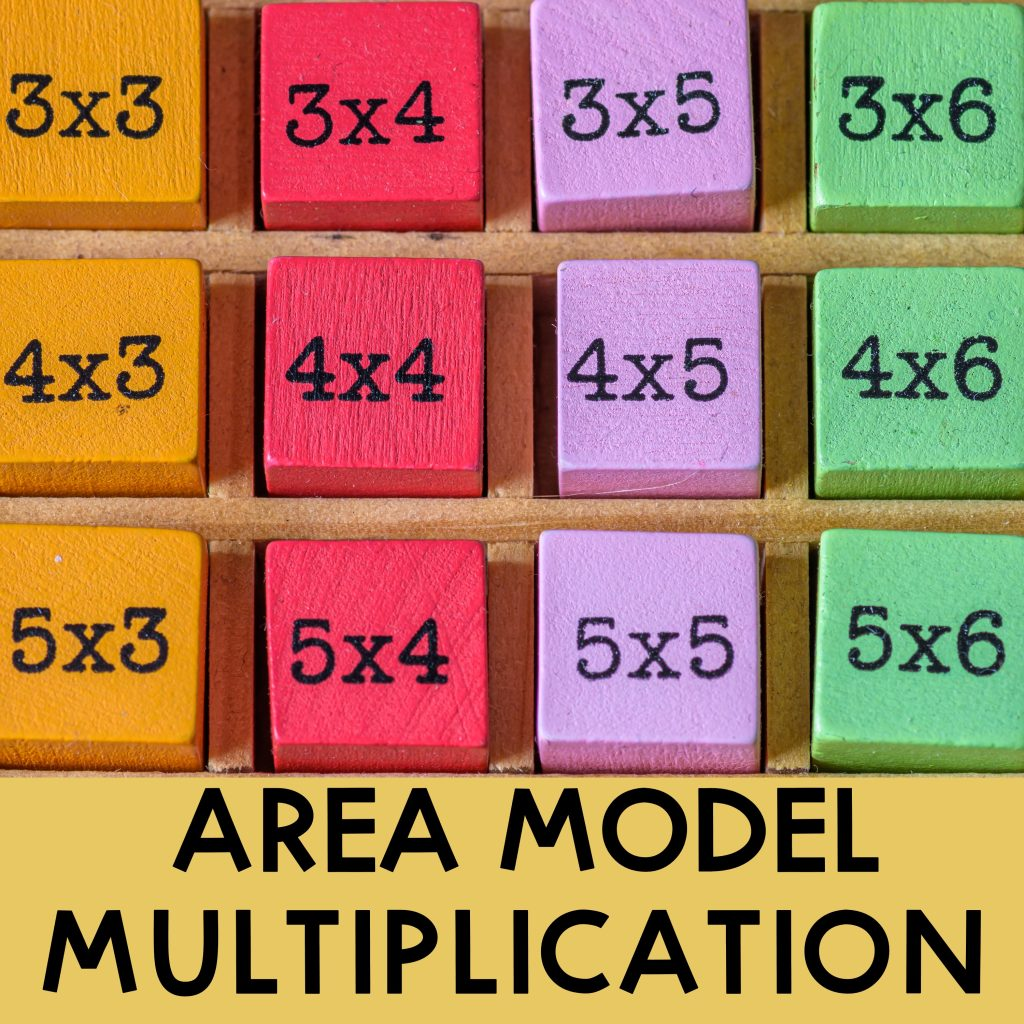## Area Model Multiplication: Guide and Examples

Area model multiplication is a great strategy when learning multi-digit multiplication. They help break down the numbers into easy-to-multiply digits and make multiplying friendly. In this article, you will learn how to use an area model to multiply 2-, 3-, and 4-digit numbers by 1-digit numbers and 2-digit numbers by 2-digit numbers. Area Model Multiplication: …